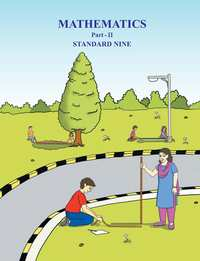SSC (English Medium) Class 9Maharashtra State Board
Share

# Balbharati Solutions for Class 9

## Solutions for Balbharati Class 9 Mathematics 2 Geometry

Shaalaa provides Balbharati Solutions for Class 9 and has all the answers for the questions given in Balbharati Class 9 Mathematics 2 Geometry. Shaalaa is surely a site that most of your classmates are using to perform in exams.

You can solve Balbharati Class 9 Mathematics 2 Geometry and use Shaalaa Balbharati Solutions for Class 9 to check your answers.

## Balbharati Solutions for Class 9

Given below is chapter wise list for Balbharati Solutions for Class 9 . Select any chapter number to view solutions.

#### Balbharati Solutions for Class 9## Chapters covered in Balbharati Solutions for Class 9

#### Chapter 1: Basic Concepts in Geometry

Shaalaa has a total of 64 questions are solved for this Chapter in Class 9 . Chapters covered in Basic Concepts in Geometry are Basic Concept in Geometry, Basic concept of segment, ray and line, Conditional Statements and Converse, Co-ordinates of points and distance, Proofs

#### Chapter 2: Parallel Lines

Shaalaa has a total of 23 questions are solved for this Chapter in Class 9 . Chapters covered in Parallel Lines are Concept for Properties of Parallel Lines with Transversal, Concept of Parallel Line, Test for Parallel Line, Use of properties of parallel lines

#### Chapter 3: Triangles

Shaalaa has a total of 45 questions are solved for this Chapter in Class 9 . Chapters covered in Triangles are Angle bisector theorem, Concept of Triangles, Congruence of Triangles, Isoscles Triangle Theorem, Median of a Triangle, Perpendicular bisector Theorem, Properties of inequalities of sides and angles of a triangle, Property of 30-60-90 Triangle Theorem, Similar Triangle, Theorem of remote interior angles of a triangle

#### Chapter 4: Constructions of Triangles

Shaalaa has a total of 14 questions are solved for this Chapter in Class 9 . Chapters covered in Constructions of Triangles are Construction of Triangles, Perpendicular bisector Theorem

Shaalaa has a total of 40 questions are solved for this Chapter in Class 9 . Chapters covered in Quadrilaterals are Concept of Quadrilaterals, Concept of Trapezium, Parallelogram, Properties of Rectangle, Properties of Rhombus, Properties of Square, Tests for parallelogram, The Mid-point Theorem

#### Chapter 6: Circle

Shaalaa has a total of 26 questions are solved for this Chapter in Class 9 . Chapters covered in Circle are Circumcircle of circle, Concept of Circles, Incircle Of a triangle, Properties of Chord

#### Chapter 7: Co-ordinate Geometry

Shaalaa has a total of 34 questions are solved for this Chapter in Class 9 . Chapters covered in Co-ordinate Geometry are Cartesian System, Equations of Lines Parallel to the X-axis and Y-axis, Graph of a Linear Equations, Introduction of Coordinate Geometry, To Plot the Points of Given Co-ordinates

#### Chapter 8: Trigonometry

Shaalaa has a total of 23 questions are solved for this Chapter in Class 9 . Chapters covered in Trigonometry are Important Equation in Trigonometry, Introduction to Trigonometry, Relation among trigonometric ratios, Terms related to right angled triangle, Trigonometric Ratios of an Acute Angle of a Right-angled Triangle, Trigonometry Table

#### Chapter 9: Surface Area and Volume

Shaalaa has a total of 34 questions are solved for this Chapter in Class 9 . Chapters covered in Surface Area and Volume are Area and Volume of Solids - Cone, Area and Volume of Solids - Sphere, Surface Area and Volume of Cube, Cuboid and Cylinder

## Balbharati Solutions for Class 9

Class 9 Balbharati solutions answers all the questions given in the Balbharati textbooks in a step-by-step process. Our Maths tutors have helped us put together this for our Class 9 Students. The solutions on Shaalaa will help you solve all the Balbharati Class 9 Geometry questions without any problems. Every chapter has been broken down systematically for the students, which gives fast learning and easy retention.

Shaalaa provides free Balbharati solutions for Class 9 . Shaalaa has carefully crafted Balbharati solutions for Class 9 Geometry that can help you understand the concepts and learn how to answer properly in your board exams. You can also share our link for free Class 9 Geometry Balbharati solutions with your classmates.

If you have any doubts while going through our Class 9 Geometry Balbharati solutions, then you can go through our Video Tutorials for Geometry. The tutorials should help you better understand the concepts.

S